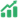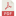# STUDENTS' MATHEMATICAL LITERACY BASED ON COVID-19 CONTEXT

• Mayang Purbaningrum Universitas Negeri Surabaya
• janet Trineke Manoy

### Abstract

Mathematical literacy is a person's ability to formulate, apply, and interpret mathematics in everyday life. This descriptive qualitative research aims to describe the mathematical literacy of 9th grade students in solving Covid-19 context problems. Four students were selected using purposive sampling techniques based on high and middle mathematical abilities. Data collection is done through math literacy tests and interviews. Research shows that students with high math skills can fulfill the mathematical literacy indicators of the stage of formulating, applying, and interpreting, with the correct answers. Middle math ability students can only fulfill the mathematical literacy indicators of the formulating stage and have not been able to answer correctly. High and middle mathematical ability students have very good abilities in formulating a problem. High mathematical ability students have excellent abilities in applying their knowledge to solve problems, while middle mathematical ability students have less ability to apply their knowledge. High mathematical ability students have sufficient ability to interpret the results obtained, while middle mathematical ability students have less ability to interpret the results obtained. High math ability students can use the information about Covid-19 provided to solve problems in the real world. Middle math ability students have not been able to use the information about Covid-19 provided to solve problems in the real world. Teachers need to provide math literacy problems on a regular basis to train students in solving everyday problems.

Published
2022-01-10
Section
ArticlesAbstract View: 71PDF_26-35 Download: 36